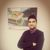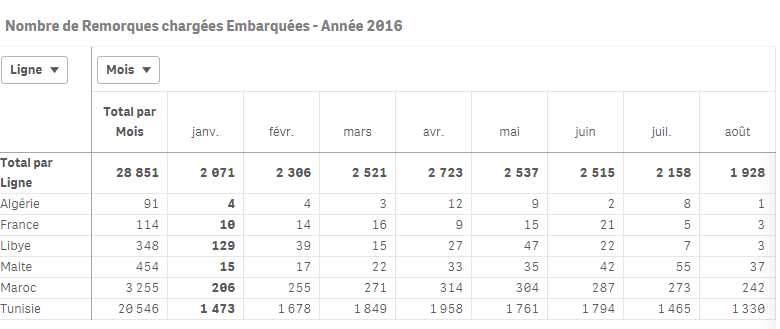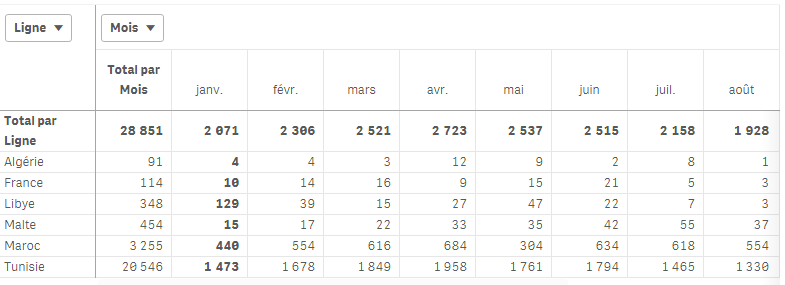# Deployment & Management

cancel
Showing results for
Did you mean:Specialist

## error in code

Hello,

i have something wrong in this code :

left join(tmp)

Pays,

Langitude,

Latitude,

Code_Ligne

FROM ///

(ooxml, embedded labels, table is Feuil1);

tmp_targa:

Where WildMatch(COMMESSA, 'AU*')

;

SELECT COMMESSA

FROM ///

[COM_BI_Dossier_Cat]:

autonumber( date(date_dossier, 'DD/MM/YYYY'),'%Date ID')  as [%Date ID]

,CodAttivita as Code_Activite,

ACTIVITE as Designation_Activite,

sens as Sens,

FilialeInserimento as Filiale_Creation_Dossier,

FilOrigine as [Filiale Origine],

PaysOrigine as [Pays Fililale Origine],

FilialeDestino as [Filiale Destination],

Code_Ligne as Code_Ligne,

Dossier ,

ViaggioNave as Num_Voyage,

date(date_dossier,'DD/MM/YYYY') as Date_Dossier,

DateEmbarquement as Date_Embarquement,

DateDebarquement as Date_Debarquement,

VideCpltGrpg as Groupe_Complet_Vide,

Plaque,

Parc,

TypeMateriel as Type_Materiel,

DesCompagnia as Compagnie,

Remorque as Remorque_Parc_HorsParc,

// if(Code_Ligne='MAR' and Date_Embarquement )

ViaggioLinea as Voyage_Ligne,

// ViaggioNave as Voyage_bateau,

carrier as Code_Carrier

,Pays,

geomakepoint(Latitude,Langitude) as PAYS2

resident tmp  Where NOT Exists(Dossier_tmp,Dossier);

drop table tmp;

final:

load * Resident [COM_BI_Dossier_Cat] where (upper(Groupe_Complet_Vide)='V');

load * Resident [COM_BI_Dossier_Cat] where (upper(Groupe_Complet_Vide)<>'V');

drop table [COM_BI_Dossier_Cat];

NoConcatenate

Fact_Final:

Plaque,

if( Nombre_Carrier>1, 1, 0) as Flag_Doublon;

Date_Embarquement,

Plaque,

count(Code_Carrier) as Nombre_Carrier

Resident final

Where Code_Ligne = 'MAR'

Group by Date_Embarquement,Plaque

;

join(Fact_Final)

Resident final

Where Code_Ligne = 'MAR'  ;

when i drop table final i have just informations of the Fact_Final,when i drop final i have just data of Fact Final what i should do ?

Thanks

1 Solution

Accepted SolutionsSpecialist
Author

Thank you Sunny for your help the solution was

if (Only({<[Month of date]>}Pays)='MAR',

9 RepliesSpecialist
AuthorMVP

May be try this

.

.

.

[COM_BI_Dossier_Cat]:

autonumber( date(date_dossier, 'DD/MM/YYYY'),'%Date ID')  as [%Date ID]

,CodAttivita as Code_Activite,

ACTIVITE as Designation_Activite,

sens as Sens,

FilialeInserimento as Filiale_Creation_Dossier,

FilOrigine as [Filiale Origine],

PaysOrigine as [Pays Fililale Origine],

FilialeDestino as [Filiale Destination],

Code_Ligne as Code_Ligne,

Dossier ,

ViaggioNave as Num_Voyage,

date(date_dossier,'DD/MM/YYYY') as Date_Dossier,

DateEmbarquement as Date_Embarquement,

DateDebarquement as Date_Debarquement,

VideCpltGrpg as Groupe_Complet_Vide,

Plaque,

Parc,

TypeMateriel as Type_Materiel,

DesCompagnia as Compagnie,

Remorque as Remorque_Parc_HorsParc,

// if(Code_Ligne='MAR' and Date_Embarquement )

ViaggioLinea as Voyage_Ligne,

// ViaggioNave as Voyage_bateau,

carrier as Code_Carrier

,Pays,

geomakepoint(Latitude,Langitude) as PAYS2

resident tmp  Where NOT Exists(Dossier_tmp,Dossier);

drop table tmp;

Fact_Final:

'Vide' as Charge_Vide

Resident [COM_BI_Dossier_Cat]

Where Upper(Groupe_Complet_Vide) = 'V';

Concatenate (Fact_Final)

'Chargé' as Charge_Vide

Resident [COM_BI_Dossier_Cat]

Where Upper(Groupe_Complet_Vide) <> 'V';

DROP Table [COM_BI_Dossier_Cat];

Left Join (Fact_Final)

Plaque,

If(Nombre_Carrier > 1, 1, 0) as Flag_Doublon;

Plaque,

Count(Code_Carrier) as Nombre_Carrier

Resident Fact_Final

Where Code_Ligne = 'MAR'

Group by Date_Embarquement, Plaque;

See if the above script works.....Specialist
Author

Thanks i find a solutionMVP

What was the solution? Can you share that with us?

Thanks,

SunnySpecialist
Author

actually this is the problem of the other problem posted,so it's not resolved and i tryed you code but it doesn't work what i can doPartner

Actually Sunny, we're trying to solve the same problem (I shared with friday) in a different way:

This is how we altered the script:

Fact_Final:

Plaque,

if( Nombre_Carrier>1, 1, 0) as Flag_Doublon;

Date_Embarquement,

Plaque,

count(Code_Carrier) as Nombre_Carrier

Resident final

Where Code_Ligne = 'MAR'

Group by Date_Embarquement,Plaque

;

join(Fact_Final)

Resident final

Where Code_Ligne = 'MAR'  ;

Concatenate

load * resident final where Code_Carrier <>'MAR';

drop table final;

Now, in the presentation, we created a table like this :

As dimension; code_ligne , as column month of dates

and the expression is :

if (Code_Ligne='MAR',if (

Mod(count( distinct {<[Year of date]={\$(=max([Year of date]))}, [Month of date]=,Timeframe='Annuel',Code_Ligne={'MAR'},Groupe_Complet_Vide={C,G},Flag_Doublon={1}>} Plaque),2)=1,

(

Count({<[Year of date]={\$(=max([Year of date]))},Code_Ligne={'MAR'}, [Month of date]=,Timeframe='Annuel',Groupe_Complet_Vide={C,G},Flag_Doublon={1}>}Dossier)

/

count(  {<[Year of date]={\$(=max([Year of date]))},Code_Ligne={'MAR'}, [Month of date]=,Timeframe='Annuel',Groupe_Complet_Vide={C,G},Flag_Doublon={1}>} Plaque)

)

+

Count({<[Year of date]={\$(=max([Year of date]))},Code_Ligne={'MAR'},[Month of date]=,Timeframe='Annuel',Groupe_Complet_Vide={C,G},Flag_Doublon={0}>}Dossier),

(

Count({<[Year of date]={\$(=max([Year of date]))},[Month of date]=,Code_Ligne={'MAR'},Timeframe='Annuel',Groupe_Complet_Vide={C,G},Flag_Doublon={1}>}Dossier)

/

count( distinct {<[Year of date]={\$(=max([Year of date]))},[Month of date]=,Code_Ligne={'MAR'},Timeframe='Annuel',Groupe_Complet_Vide={C,G},Flag_Doublon={1}>} Plaque)

)

+

Count({<[Year of date]={\$(=max([Year of date]))},[Month of date]=,Timeframe='Annuel',Code_Ligne={'MAR'},Groupe_Complet_Vide={C,G},Flag_Doublon={0}>}Dossier)

),

Count({<[Year of date]={\$(=max([Year of date]))}, [Month of date]=,Groupe_Complet_Vide={C,G}>}Dossier))

Now, the table is as follow and all the values are correct :Now, if I choose a month, the values mustn't change at all : BUT when  I do, only the values of Ligne Maroc change (wrong values), only the month selected returns a correct value(iin this case, I selected Month : Mai)This is what I can't understand.

So stalwar1‌, if you can understand what could be causing this, your help would be much appreciated, as always !Specialist
Author

Hello stalwar1‌ do you have any solution for this ?MVP

Would you be able to email me the application? I can take a look once I am homeSpecialist
Author

Thank you Sunny for your help the solution was

if (Only({<[Month of date]>}Pays)='MAR',